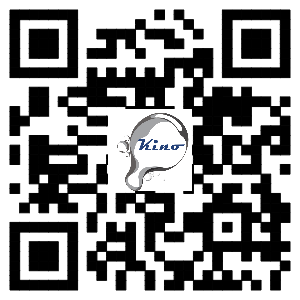﻿ What is the modern contact angle meter?
Welcome to the USA KINO: The world leading supplier of contact angle meter, surface & interfacial tensiometer &TOF camera
##### Position
What is the modern contact angle meter?
2019-2-15 13:33:58

At presen, you can easily find a contact angle meter for measuring contact angle between the solid and liquid. Among them, some instruments are the real one which is used for measuring contact angle, but others are not.

1, Contact angle meter based on Circle Fitting method and Ellipse, Tangent method is just a Goniometer.

Most instruments of the commercialized contact angle meters use Circle Fitting, Ellipse Fitting or Tangent Fitting method. These methods worked until there is a better method to characterize the physicochemical property of the solid. These methods cannot correct the effect of the gravity, surface tension, contact angle, interface tension between the solid and liquid, and just measure the angle of the geometric curve. This doesn't make any physical chemistry sense. Instruments with geometric curve fitting method cannot tell you more. And the result of the contact angle may also mislead you, they have no repeatability and comparison.

(1)    Gravity will affect the value of contact angle even if you dose a drop with volume 2uL.

(2)    Ellipse fitting method cannot correct the effect of gravity. And it can only fit 80% drop shape not all.

(3)    Contact angle is the physicochemical property of the solid and it’s more than an angle of curve.

2, the history of the contact angle meter.

In the 1950s, Pro. Zisman made the first contact angle goniometer to measure contact angle of the solid. They used a reticle in the microscope and rotated the reticle manually to get the value of contact angle.( W.C.Bigelow, D.L.Pickett.W.A.J.Zisman, Coll.Sci, 1,513 (1946))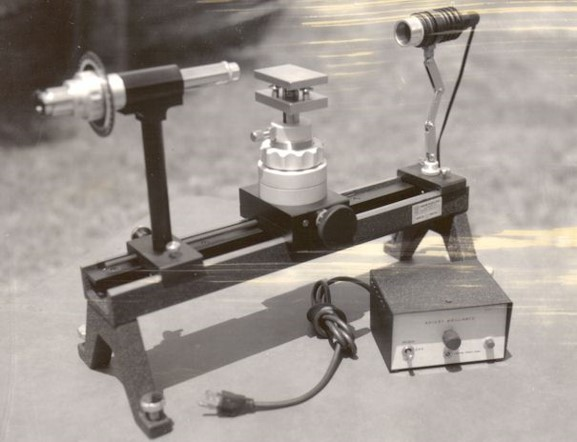In the end of 1980s, With the development of computer technology, commercialized contact angle meter used the image analysis technique to measure the contact angle by capturing the drop image, finding the drop edge from captured image, then fitting the drop profile by the Circle, Ellipse, Tangent (polynomial, composite type equation or spline) curve. Now, most commercialized contact angle meter still regards these methods as the main or only methods to measure contact angle. So, they are just a goniometer and not the real sense of contact angle meter.

In the end of 1980s, Pro. A.W.Neumann proposed a new method named ADSA-P to measure surface tension of the liquid or interface tension between the liquid and fluid. They used the Young-Laplace Equation which included the surface tension, interface tension and the gravity coefficient, and contact angle. But they used the ADSA-P method mostly for measuring surface tension or interface tension, not mainly for measuring contact angle. And the assumption of axisymmetric liquids limited the application of ADSA-P in the measurement of contact angle.(Rotenberg, Y., Boruvka, L. and Neumann, A.W. Determination of Surface Tension and Contact Angle from the Shapes of Axisymmetric Fluid Interfaces J. Colloid Interface Sci. 93 p.169-183）)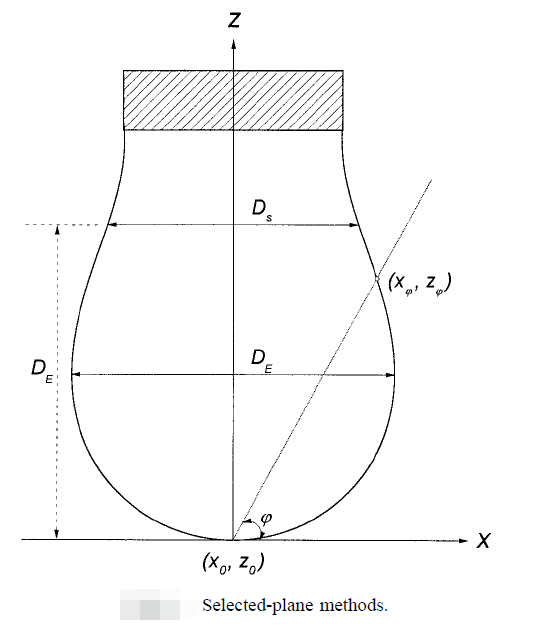In the 1990s, some commercialized contact angle meters made in German provide the Young-Laplace Equation fitting method to measure contact angle. The Young-Laplace Equation fitting method is used for measuring contact angle more than 120°, these machines still regard the circle fitting or ellipse fitting method as the main method to measure contact angle, because Young-Laplace equation method can only measure contact angle of axisymmetric drop. But the truth is drop shape of 98% surface of solid is asymmetric due to surface roughness, chemical diversity and heterogeneity of surface structure.So, ADSA-P or the Young-Laplace equation fitting method is the real method for measuring contact angle, and the result from it is more acceptable than the geometric curve fitting method. And the assumption of axisymmetric liquids limited the application of ADSA-P in the measurement of contact angle.

3, The truth that we have to accept: 98% surface of solid is asymmetric.

You cannot find out a perfect circle drop from the top view of the drop shapes at the front windshield. And the same, more than 98% surfaces of solid have irregular drop shape.So, the most effective and practical method is measuring contact angle of solid surface based on axisymmetric drop.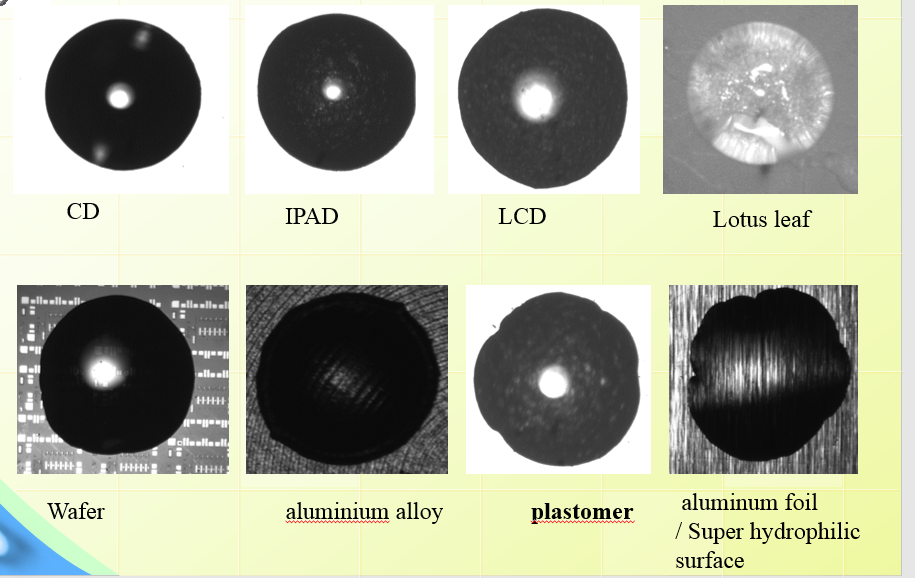4, ADSA-RealDrop / TrueDrop is what you need for measuring contact angle between solid and liquid.

ADSA-Realdrop /TrueDrop based on ADSA-NA, correcting the effect of gravity, measures the axisymmetric drop shape of solid. And we will provide you the equilibrium contact angle based on Tadmor method. (At the drop image shown below, left contact angle is 155.557 and right contact angle is 146.842)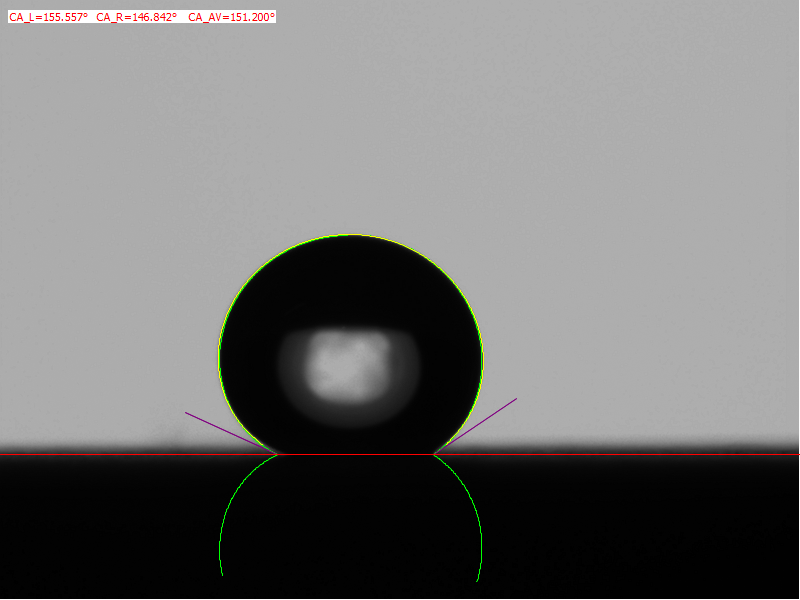(1)3D contact angle,

(2) the cleanness of the surface by just one drop.

(3) homogeneity of your sample ( A difference value than 2°from the left and right contact angle means your sample is not homogeneity)

(4) design special surface like rice leaf or bamboo leaf. So that the drop can only roll off by one direction).

Only ADSA-RealDrop can guarantee a real contact angle meter. Unlike the Young-Laplace Equation fitting method can only be used to measure 2% axisymmetric drop shape, ADSA-RealDrop method is the only method which can be used to measure 100% surface of solid.

5, Technical route of the 3D contact angle

(1)The most simple way to measure 3D contact angle:  Rotating the sample by 360° horizontal direction (The Patent of KINO)

(2) Using the 360° lens to capture the drop images by different view directions. (The patent of KINO)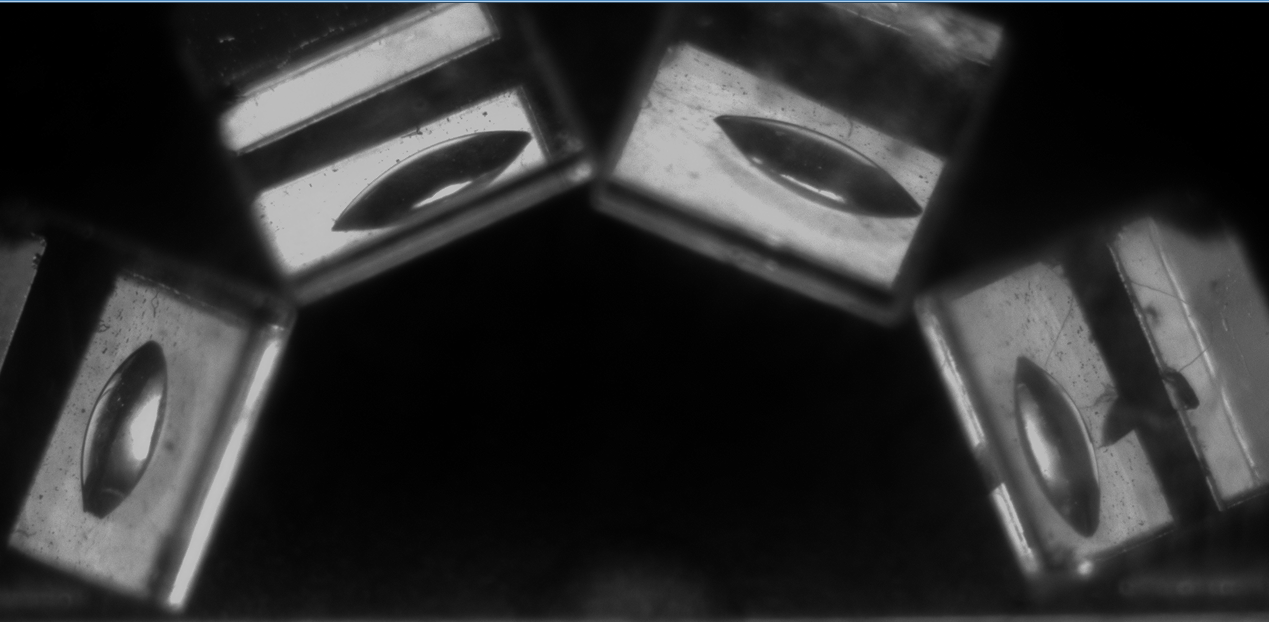(3) 3D camera such as TOF camera

6, A sample of measuring contact angle by various method:

A  contact angle chromatograms of setaria viridis leaf.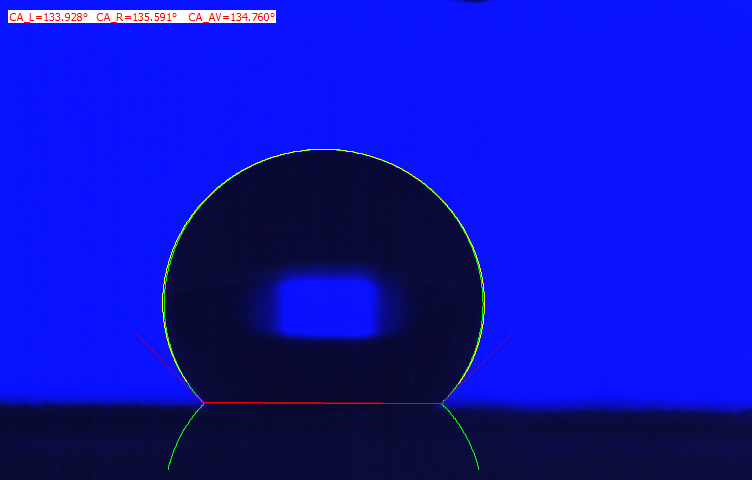(1) When it's perpendicular to the lens, the contact angle chromatograms of setaria viridis leaf as shown below. We can find the microvilli of the leaf.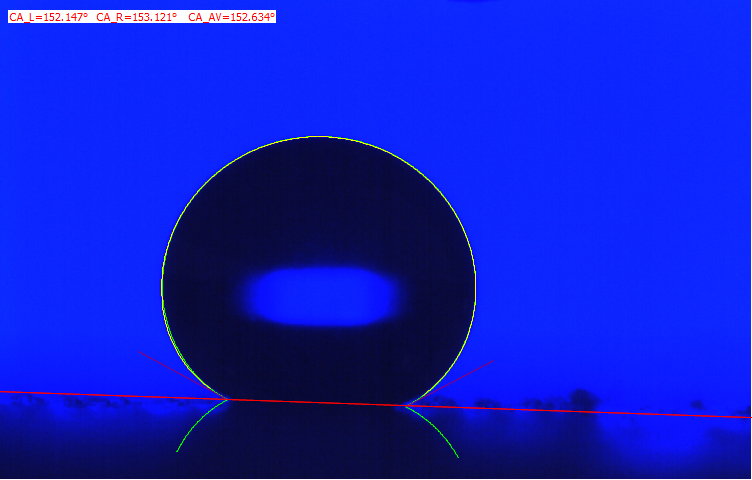The advanced and receded contact angle of the setaria viridis leaf .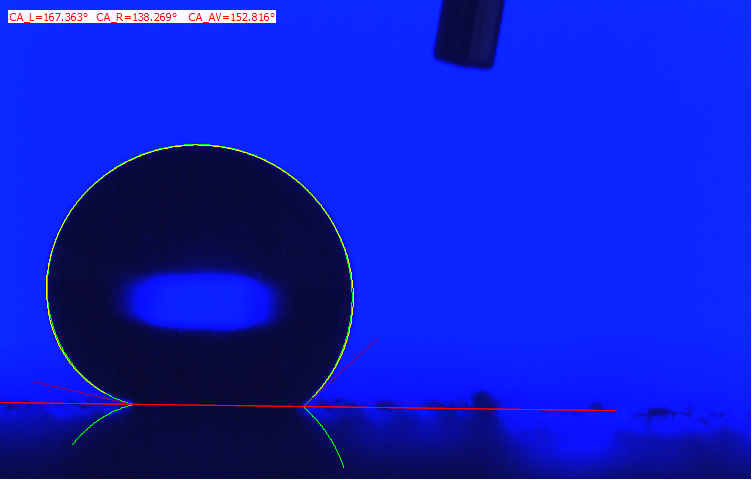If you try to fiiting the same drop shape with other methods, you will not get the same acceptable result as the ADSA-RealDrop

(1) Young-Laplace equation fitting method for measuring contact angle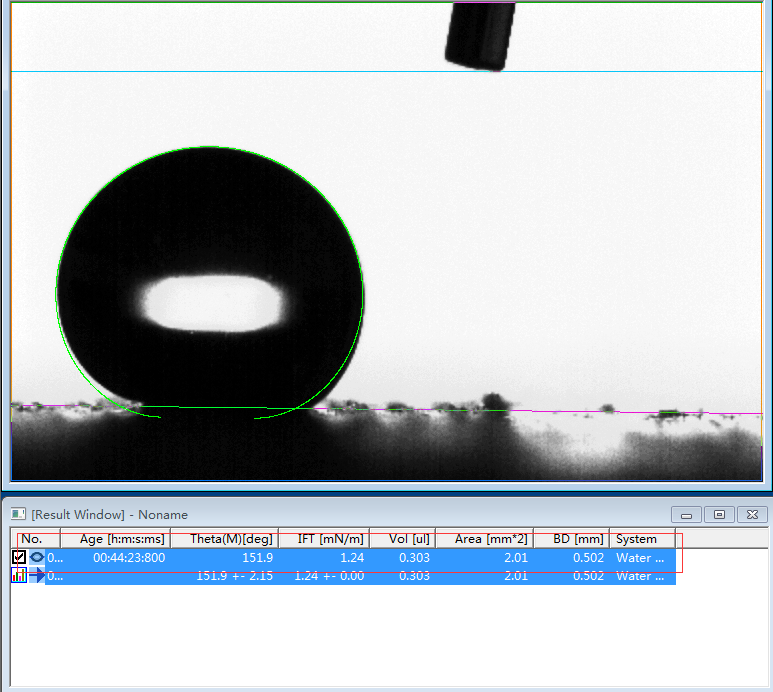(2) Ellipse fittting method for measuring contact angle.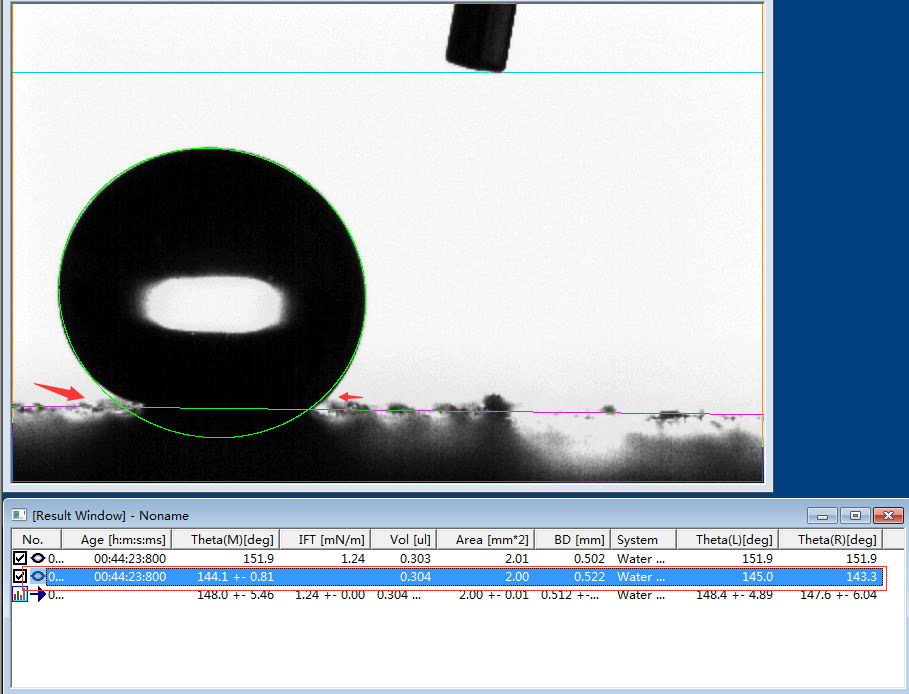##### Focus news